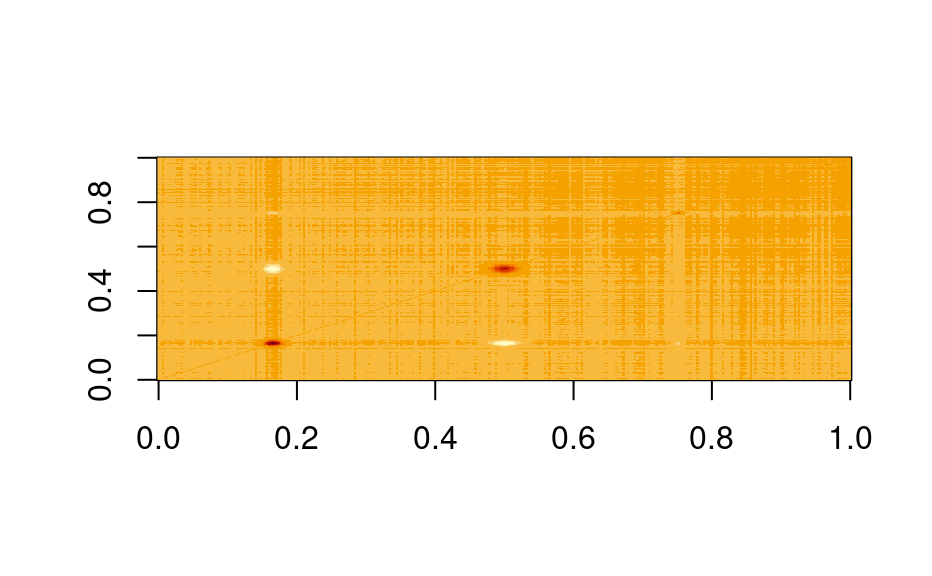Covariance matrices for hyperSpec objects

# S4 method for hyperSpec,missing
cov(
x,
y = NULL,
use = "everything",
method = c("pearson", "kendall", "spearman")
)

cov_pooled(x, groups, ..., regularize = NULL)

## Arguments

x

hyperSpec object.

y

Not supported.

use, method

handed to stats::cov()

groups

Factor indicating the groups.

...

Ignored.

regularize

(numeric): Regularization of the covariance matrix. Set 0 to switch off. Default is 1e-5 * max(abs(cov_p)), where cov_p is a pooled covariance matrix before regularization.

cov_pooled() calculates pooled covariance like, e.g., in LDA.

## Value

Covariance matrix of size nwl(x) x nwl(x).

stats::cov()

C. Beleites

## Examples

image(cov(faux_cell))pcov <- cov_pooled(faux_cell, faux_cell$region) plot(pcov$means)image(pcov\$COV)